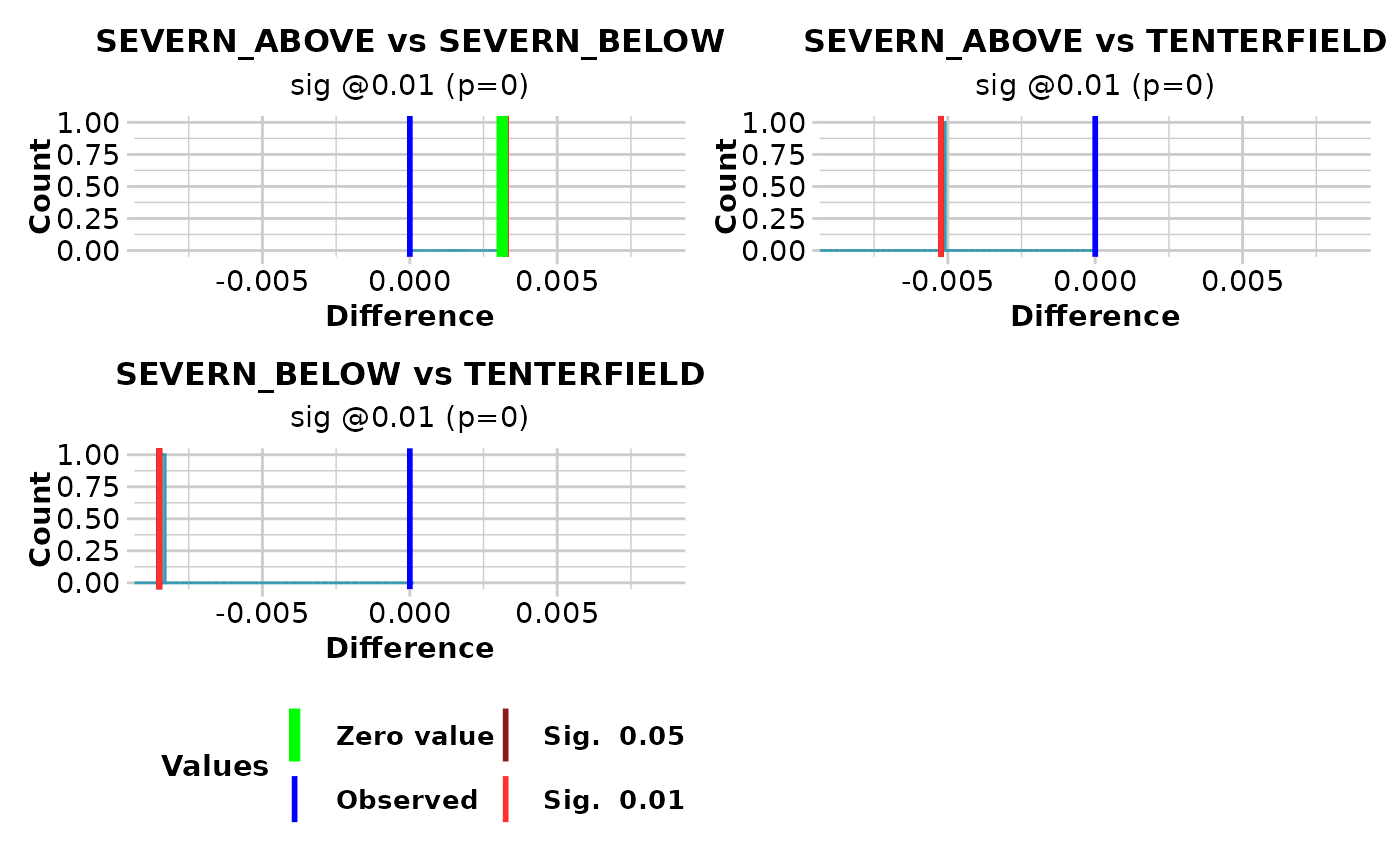Calculates the expected heterozygosities for each population in a genlight object, and uses re-randomization to test the statistical significance of differences in heterozygosity between populations taken pairwise.

## Usage

gl.test.heterozygosity(
x,
nreps = 100,
alpha1 = 0.05,
alpha2 = 0.01,
plot.out = TRUE,
max_plots = 6,
plot_theme = theme_dartR(),
plot_colors = two_colors,
save2tmp = FALSE,
verbose = NULL
)

## Arguments

x

A genlight object containing the SNP genotypes [required].

nreps

Number of replications of the re-randomization [default 1,000].

alpha1

First significance level for comparison with diff=0 on plot [default 0.05].

alpha2

Second significance level for comparison with diff=0 on plot [default 0.01].

plot.out

If TRUE, plots a sampling distribution of the differences for each comparison [default TRUE].

max_plots

Maximum number of plots to print per page [default 6].

plot_theme

Theme for the plot. See Details for options [default theme_dartR()].

plot_colors

List of two color names for the borders and fill of the plots [default two_colors].

save2tmp

If TRUE, saves any ggplots and listings to the session temporary directory (tempdir) [default FALSE].

verbose

Verbosity: 0, silent or fatal errors; 1, begin and end; 2, progress log; 3, progress and results summary; 5, full report [default NULL, unless specified using gl.set.verbosity].

## Value

A dataframe containing population labels, heterozygosities and sample sizes

## Details

Function's output

If plot.out = TRUE, plots are created showing the sampling distribution for the difference between each pair of heterozygosities, marked with the critical limits alpha1 and alpha2, the observed heterozygosity, and the zero value (if in range).

Plots and table are saved to the temporal directory (tempdir) and can be accessed with the function gl.print.reports and listed with the function gl.list.reports. Note that they can be accessed only in the current R session because tempdir is cleared each time that the R session is closed.

Examples of other themes that can be used can be consulted in

## Author

Custodian: Luis Mijangos (Post to https://groups.google.com/d/forum/dartr)

## Examples

out <- gl.test.heterozygosity(platypus.gl, nreps=1, verbose=3, plot.out=TRUE)
#>   Processing genlight object with SNP data
#>   Warning: data include loci that are scored NA across all individuals.
#>   Consider filtering using gl <- gl.filter.allna(gl)
#>   Warning: genlight object contains monomorphic loci which will be factored into heterozygosity estimates
#>
#>
#> Starting gl.test.heterozygosity
#>
#>   Calculating the sampling distributions for pairwise differences between populations by re-randomization
#>   Please be patient .... go have a coffee
#>   Cycling through the 3 pairs of populations
#>   Plotting sampling distributions
#>#>           pop1         pop2      diff significance pval
#> 1 SEVERN_ABOVE SEVERN_BELOW  0.003134    sig @0.01    0
#> 2 SEVERN_ABOVE  TENTERFIELD -0.009777    sig @0.01    0
#> 3 SEVERN_BELOW  TENTERFIELD -0.012911    sig @0.01    0
#> Completed: gl.test.heterozygosity
#>